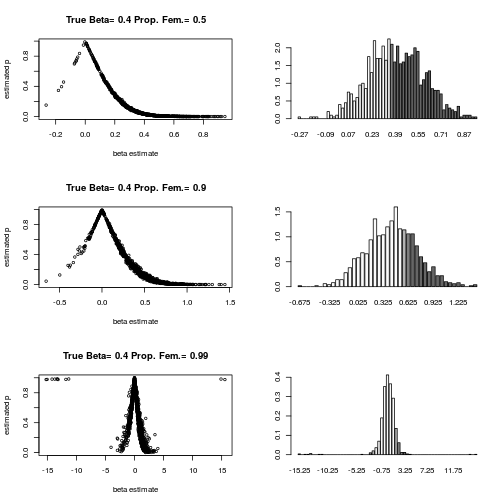``````### Paul Johnson <pauljohn@ku.edu>
### 2010-06-16, cleanup 2014-08-05

### Question arose in the categorical analysis class today.

### If one fits a logistic regression to estimate the effect of, say
### sex, what "goes wrong" if the numbers of men and women in a sample
### becomes "unbalanced".

### So I spent the afternoon writing this down.  Please run it, then
### look at the figure. The "true beta" for sex is 0.4.

### In the plot on the left, you see the estimates of beta plotted
### against the reported p values for the hypothesis test that the
### Null hypothesis that beta=0. On the right side, you see the
### histogram for the sampling distribution of 1000 runs.
### The color coding of the bars represents the proportion of beta
### estimates in that range which are judged 'statistically
### significant' at the 0.05 level.

### What do I see when I look at this?

### People who say "don't report betas unless they have p < 0.05
### are doing a lot of damage to our body of knowledge. Here, you
### know the "True" beta is 0.4, the estimates for balanced samples
### are around 0.4, but if you only look at the ones with small
### p values, you are creating a very biased picture for yourself.

### Second, as long as the imbalance between men and women is not
### worse than 90% to 10%, the beta estimates are not too horribly
### affected.  Of course, the best estimates (in terms of efficiency)
### are obtained with the split is 50-50.
### pj

N <- 1000
A <- -1
B <- 0.3

x <- 1 + 10 * rnorm(N)
myeta <- A + B * x

simLogit <- function(myeta){
myN <- length(myeta)
mypi <- exp(myeta)/(1+exp(myeta)) ## SAME AS 1/(1+exp(-myeta))
myunif <- runif(myN)
y <- ifelse(myunif < mypi, 1, 0)
}

y <- simLogit(myeta)

##plot(x,y, main=bquote( eta[i] == .(A) +   .(B) * x[i] ))

###text ( 0.5*max(x), 0.5, expression( Prob( y[i] == 1) == frac( 1 , 1 + exp(-eta[i] ))))

### This calculates just one logistic regression
myglm1 <- glm ( y ~ x, family = binomial(link = "logit") )
summary(myglm1)
``````
``````##
## Call:
## glm(formula = y ~ x, family = binomial(link = "logit"))
##
## Deviance Residuals:
##    Min      1Q  Median      3Q     Max
## -2.856  -0.544  -0.138   0.502   3.373
##
## Coefficients:
##             Estimate Std. Error z value Pr(>|z|)
## (Intercept)   -1.036      0.108   -9.61   <2e-16 ***
## x              0.297      0.019   15.66   <2e-16 ***
## ---
## Signif. codes:  0 '***' 0.001 '**' 0.01 '*' 0.05 '.' 0.1 ' ' 1
##
## (Dispersion parameter for binomial family taken to be 1)
##
##     Null deviance: 1342.71  on 999  degrees of freedom
## Residual deviance:  711.53  on 998  degrees of freedom
## AIC: 715.5
##
## Number of Fisher Scoring iterations: 6
``````
``````simUnbalanced <- function(iter=0, C, PrFem){
sex <- ifelse(runif(N) < PrFem,0,1)
myeta <- A + B * x + C * sex
sex <- factor(sex, levels=c(0,1), labels=c("M","F"))
y <- simLogit(myeta)
myglm2 <- glm ( y ~ x + sex, family = binomial(link="logit") )
myglm2sum <- coef(summary(myglm2))
est <- myglm2sum[3,]
est
}

gc <- gray.colors(10)

createFigs <- function(result){
dat <- result[]
C <- result[["C"]]
PrFem <- result[["PrFem"]]
mybeta <- dat[1,]

##hist(mybeta,freq=F,breaks=50)
hrow1 <- hist(mybeta,breaks=50, plot=F)
mybreaks <- hrow1\$breaks

breakMember <- cut(dat[1,], mybreaks)

mypval <- dat[4,]

mysignif <- ifelse( (mypval < 0.05 ) , 1, 0)

df <- data.frame(mybeta, mypval, mysignif, breakMember)

propsig <- by(df\$mysignif, INDICES=list(df\$breakMember), mean, simplify = TRUE)
mytrat <- dat[3,]

mycounts <- hrow1\$counts

##plot( hrow1\$breaks[-1], hrow1\$density, type="S")

plot(dat[1,], dat[4,], xlab = "beta estimate", ylab = "estimated p",
cex = 0.7, main = paste("True Beta=", C, "Prop. Fem.=", PrFem))

gc <- gray.colors(10)

gc <- c("gray98","gray70","gray50","gray40")

cut(propsig, breaks=c(-1,0.1,0.5,0.9,1.1))

catpropsig <- cut(propsig, breaks = c(-1,0.1,0.5,0.9,1.1), ordered = TRUE,
labels = c("0","lth","mth","1"))

barplot(hrow1\$density, col=gc[as.numeric(catpropsig)], names=hrow1\$mids)
}

## TO save graphs into a file, change SAVEME to TRUE
SAVEME <- FALSE

## If you want all of the graphs in one big file, change onefile
## to TRUE
if (SAVEME) pdf(file = "logitUnbalanced-%03d.pdf", height=10,
width=7.5, paper="special", onefile = FALSE)
par(mfrow = c(3,2)) ## 3 x 2 array of graphs. Want separate pages?
# Don't run this

C <- 0.4
PrFem <- 0.5

result45 <- list(sapply(1:1000, simUnbalanced, C = C, PrFem = PrFem),
C = C, PrFem = PrFem)

createFigs(result45)

PrFem <- 0.9

result49 <- list(sapply(1:1000, simUnbalanced, C = C, PrFem = PrFem),
C = C, PrFem = PrFem)

createFigs(result49)

PrFem <- 0.99

dat499 <- list(sapply(1:1000, simUnbalanced, C = C, PrFem = PrFem), C
= C, PrFem = PrFem)

createFigs(dat499)
````````````if (SAVEME) dev.off()
``````